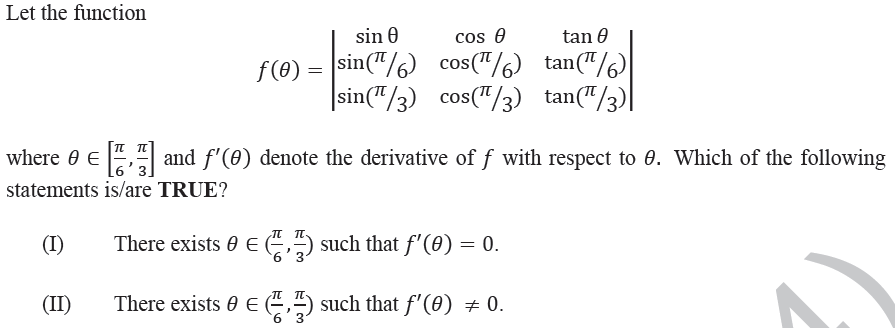# GATE | GATE-CS-2014-(Set-1) | Question 16(A) I only
(B) II only
(C) Both I and II
(D) Neither I nor II

Explanation: In the given question,ranges fromto.
When=, the value of the function is 0, since Rows 1 and 2 are the same.
When=, the value of the function is 0, since Rows 1 and 3 are the same.
Therefore,The given function is continuous and differentiable in the given range since it is a trigonometric function.
Then According to the Mean Value Theorem,
There exists a valueof such that. So the first statement is true.
Also there may also exist a value, such that. This is because the function is not a constant function, i.e. all values in the range are not equal to.
So the second statement is also true.
Therefore the correct option is C.

Quiz of this Question

My Personal Notes arrow_drop_up

Article Tags :

Be the First to upvote.

Please write to us at contribute@geeksforgeeks.org to report any issue with the above content.